# Count Cells Not Equal To in Excel & Google Sheets

This tutorial will demonstrate how to count cells not equal to using the COUNTIF Function in Excel and Google Sheets.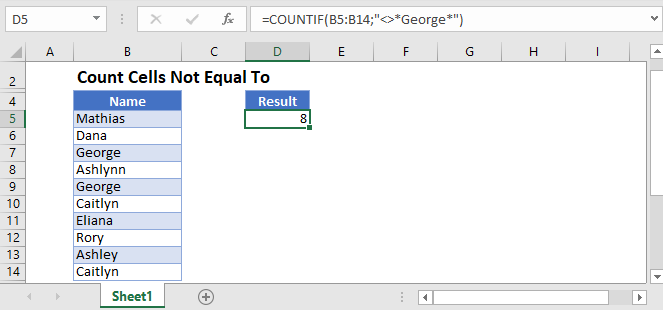## Count Cells Not Equal To with COUNTIF

The COUNTIF Function counts cells that meet certain criteria. You will be required to enter two fields: range and criteria.

In the example below, we want to count how many students have exam scores not equal to “Pass”.

``=COUNTIF(D3:D11,"<>*Pass*")``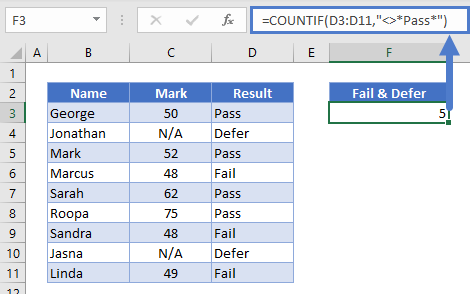The symbol “<>” in Excel/Google Sheets is equivalent to “not equal to”. In this case, we are checking how many values in the range C2:C10 are not equal to “Pass”.

You will also notice that criteria is enclosed in quotation marks. Please note that this formula will not work if you do not have the quotation marks around the criteria.

You can also use the COUNTIF function for numeric criteria. As demonstrated in the above example, if you want to check how many students scored over 50, you can use the following formula:

``=COUNTIF(C3:C11, ">"&50)``

You will notice that in this example, our criterion is a number. For this reason, we will not enclose the 50 inside of the quotation marks.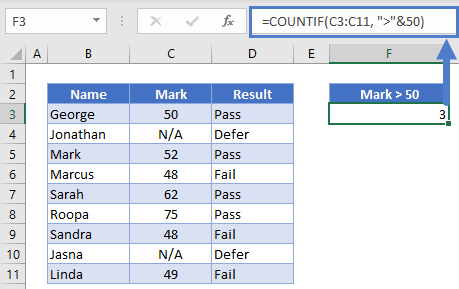You can also use the COUNTIF function to reference another cell in its criteria.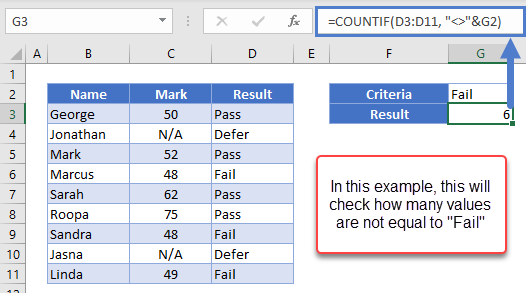In this next example, we set up Data Validation with our criterion cell.

``=COUNTIF(, “<>”&F3) [where F3 is the criteria you don't want your range to equal to]``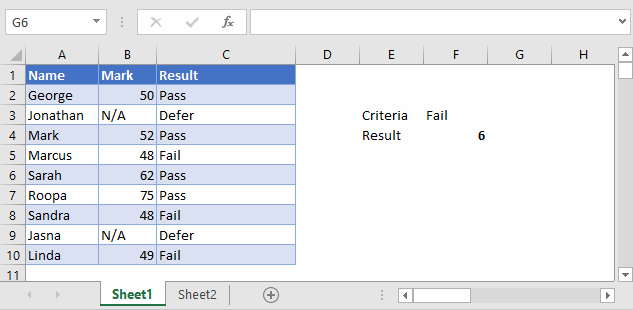You can see that Data Validation is a great tool for creating interactive dashboards.

## Count Cells Not Equal To with COUNTIF in Google Sheets

The formulas above work in the exact same way in Google Sheets as well.

To count cells not equal to certain string criteria: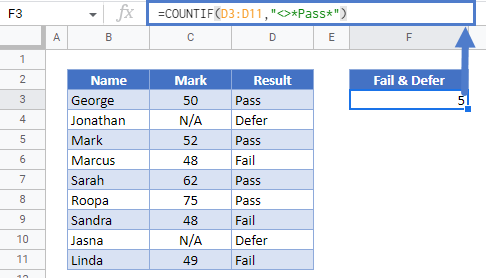To count cells not equal to/greater/less than numeric criteria: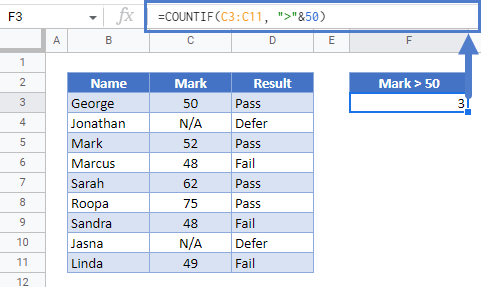### Excel Practice Worksheet

Practice Excel functions and formulas with our 100% free practice worksheets!